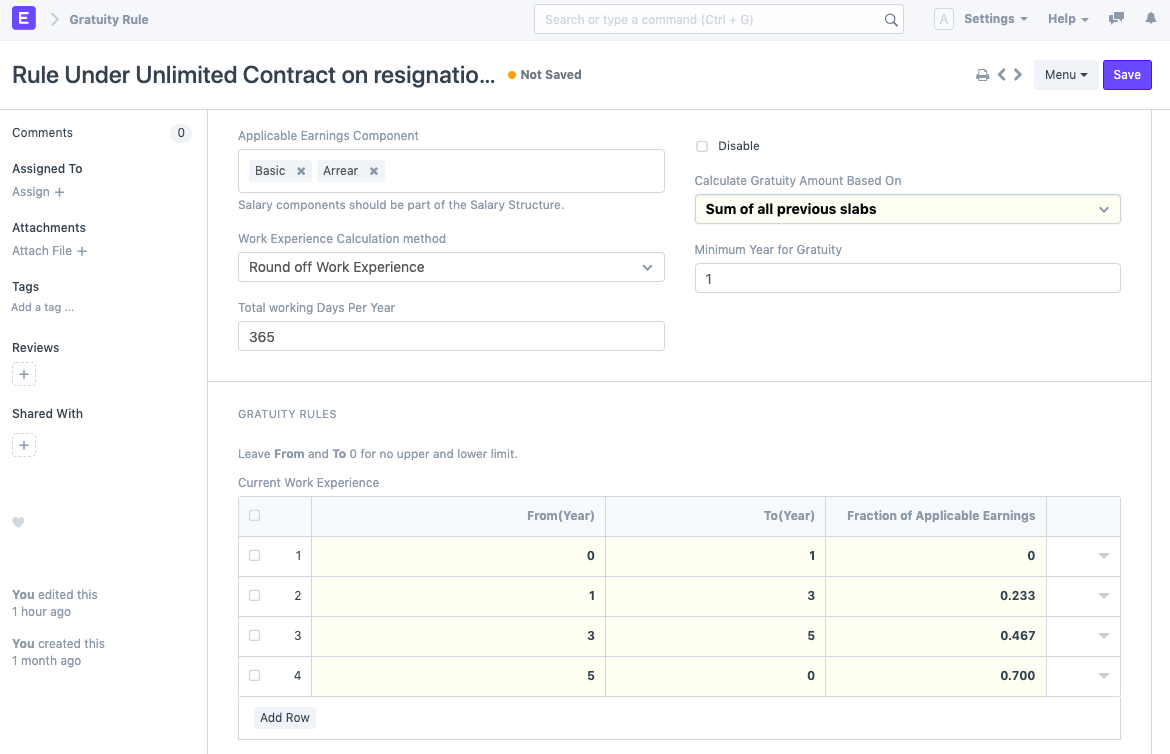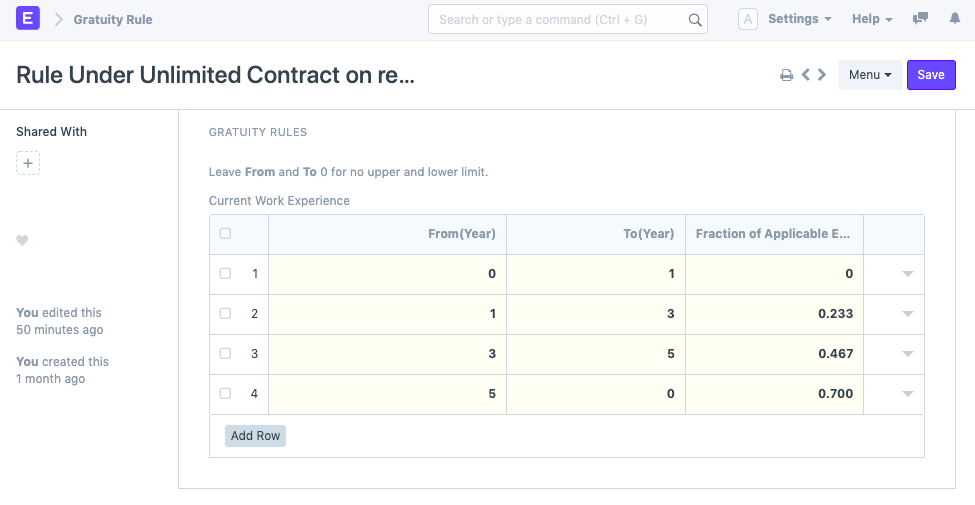# Gratuity Rule

> This Feature is introduced in Version 13, which will be part of separate Payroll Module.

Gratuity Rule are set of rule defined by Central or State used during calculation of Gratuity Amount

In Frappe HR, you can define different Gratuity Rules based on different Region.

To access the Gratuity Rule, go to:

> Home > Payroll > Gratuity Rule

## 1. Prerequisites

Before creating an Gratuity Rule, it is advised to create the following:

## 2. How to create Gratuity Rule

1. Got to Gratuity Rule > New
2. Select Applicable Components. These Salary Components contribute during Gratuity Calculation.
3. Select "Calculate Gratuity Amount based on"
4. Define Gratuity Rule
5. SaveSome of the additional attributes used while gratuity Calculation are define below.

### 3.1 Work Experience Calculation method:

Frappe HR provide two different method for calculation of Work experience.

1. Round off Work Experience method Round off yor current experience. For example, if employee have total experience of 3 year and 6 month will be treated as 4 year experience.
2. Take Exact Completed Year.

### 3.2 Calculate Gratuity Amount Based On:

Let's consider the following example to understand the calculation.1. Current slab: If Gratuity Amount calculation is based on Current Slab, then amount will be the product of Work Experience (in years), Fraction of Applicable Earnings and summation of the Applicable Earnings Components. Based on above Gratuity Rules/slab, if an employee has an experience of 5 years, then it falls in third slab. The calculation of Gratuity Amount will be as follows:

> Gratuity amount = 5 * 0.467 * (Arrear + Basic)

1. Sum of all previous slabs: If Gratuity Amount calculation is based on Sum of all previous slabs, then amount will be the summation of product of individual slabs up to the year of experience and summation of Applicable Earnings Component. Based on above Gratuity Rules/slab, if an employee has an experience of 5 years, then the calculation of Gratuity Amount will be as follows:

> Gratuity amount = [(1 * 0) + (2 * 0.233) + (2 * 0.467)]*(Arrear + Basic)